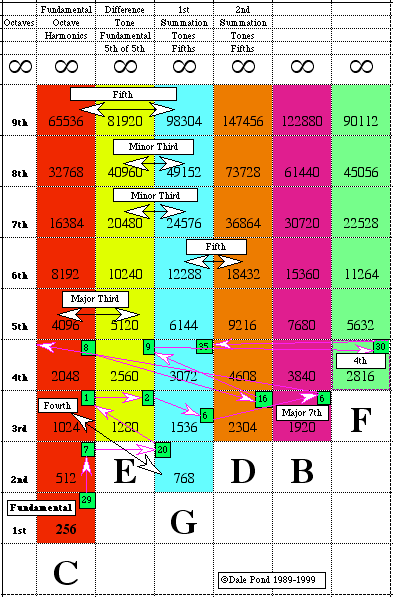# Overtone series

 Overtone SeriesOvertone Series Sympsionics Symbol

A naturally occurring series of overtones, partials or harmonics from a fundamental or any other tone, sound or frequency. The nature of this Progressive Evolution is discretely arithmetical according to what is vibrating whether it be a string, rod fastened at one end or both ends, diaphragm, volume of gas, etc. its substance, density, etc. as also by how the vibrating object is excited; striking, bowing or sympathetically. When as a volume the Progressive Evolution is geometric as in Russell's Scale of Locked Potentials.

Naturally Occurring Harmonics and Partials

Overtone Harmonics
See MUTATIONS for more complete list.
C:
First Octave
1 = c
Second Octave
2 = c'
3 = g'
Third Octave
4 = c''
5 = e''
6 = g''
7 = bb (it's actually about half way between a and bb)
Fourth Octave
8 = c'''
9 = d'''
10 = e'''
11 = f#''' (halfway between f and f#)
12 = g'''
13 = a'''
14 = bb''' (sort of)
15 = b'''
16 = c
17 = c#/db
18 = d
19 = eb (a bit flatter)
20 = e
21 = ???
22 = f# (sort of)
23 = f# (a bit sharper)
24 = g

This video contains an excellent description of overtones.Naturally Occurring Harmonics and Partials

Rod Fixed at Both Ends
"A rod fixed at both ends and caused to vibrate transversely divides itself in the same manner as a string vibrating transversely.

"But the succession of its overtones is not the same as those of a string, for while the series of tones emitted by the string is expressed by the natural numbers, 1, 2, 3, 4, 5, etc., the series of tones emitted by the rod is expressed by the squares of the odd numbers, 3, 5, 7, 9, etc." [from "Sound" by John Tyndall]

Rod Fixed at One End
"A rod fixed at one end can also vibrate as a whole, or can divide itself into vibrating segments separated from each other by nodes.

"In this case the rate of vibration of the fundamental tone is to that of the first overtone as 4:25, or as the square of 2 to the square of 5. From the first division onwards the rates of vibration are proportional to the squares of the odd numbers, 3, 5, 7, 9, etc.

"With rods of different lengths the rate of vibration is inversely proportional to the square of the length of the rod." [from "Sound", John Tyndall]

Differentiation
Figure 8.5 - Summation Tones
Harmonic
Harmonic Series
Interval
Law of Harmonic Pitch
Law of Harmonic Vibrations
Master Tone
master tones
Mid-tone
octave tones
Overtone
Overtone Position
Overtone Series
Overtones Developed Musically
Resultant Tone
Scale
Sympathetic Vibration
Sympathy
Undertone
Vibrating Rod Harmonics
1.23 - Power of Harmonics through Summation Tones
9.8 - Spontaneous Creation of Harmonic Series
9.9 - Sympathy or Harmony Between Harmonics or Overtones